# McCain Oil Formation Volume Factor equation

Jump to: navigation, search

## McCain Oil Formation Volume Factor equation

McCain equation is determined from material balance equation based on the definition of the oil formation volume factor.

## Math & Physics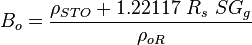$B_o = \frac{\rho_{STO}+1.22117\ R_s\ SG_g}{\rho_{oR}}$

## Example. Calculation of the oil formation volume factor

Example source 

### Input data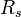$R_s$ = 53.24 sm3/sm3 at P = 10 MPa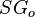$SG_o$ = 0.85 or 35 API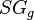$SG_g$ = 0.75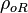$\rho_{oR}$ = 749 kg/m3 at P = 10 MPa

Calculate oil formation oil factor at p = 10 MPa?

### Solution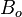$B_o$ = 1.199 rm3/sm3

The solution is available in the online PVT calculator software model at www.pengtools.com

## Application range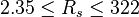$2.35 \le R_s \le 322$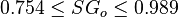$0.754 \le SG_o \le 0.989$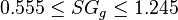$0.555 \le SG_g \le 1.245$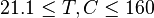$21.1 \le T, C \le 160$

## Nomenclature$B_o$ = oil formation volume factor, rm3/sm3$R_s$ = solution gas-oil ratio, sm3/sm3$SG_g$ = gas specific gravity, dimensionless$SG_o$ = oil specific gravity, dimensionless$\rho_{oR}$ = oil density at reservoir conditions, kg/m3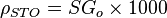$\rho_{STO} = SG_o \times 1000$ = stock tank oil density, kg/m3